# McGraw Hill Math Grade 5 Chapter 3 Lesson 5 Answer Key Multiplying by 2-Digit Whole Numbers

All the solutions provided in McGraw Hill Math Grade 5 Answer Key PDF Chapter 3 Lesson 5 Multiplying by 2-Digit Whole Numbers are as per the latest syllabus guidelines.

## McGraw-Hill Math Grade 5 Answer Key Chapter 3 Lesson 5 Multiplying by 2-Digit Whole Numbers

Multiply and then describe the strategy you used to solve each problem.

Question 1.
49 × 50 = 2450
I added 1 to 49 and multiplied 50 × 50 = 2500. Then I subtracted 50 to find the answer.

Question 2.
43 × 52 = ____
43 × 52 = 2,236.

Explanation:
Here, we have subtracted 3 from 43 and subtracted 2 from 52 multiplied by 40 × 50 = 2,000. Then added 236.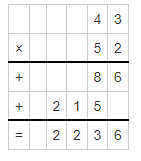Solve.

Question 3.31 × 23 = 713.

Explanation:
Here, we have subtracted 1 from 31 and 3 from 23 then multiplied 30 × 20 which is 600. Then added 113.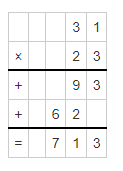Question 4.62 × 17 = 1054.

Explanation:
Here, we have subtracted 2 from 62 and added 3 to 17 then multiplied 60 × 20 which is 1200. Then subtracted 146.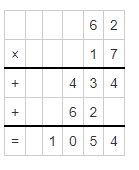Question 5.70 × 80 = 5,600.

Explanation:
By multiplying 70 × 80 we will get 5,600.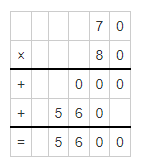Question 6.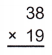38 × 19 = 78.

Explanation:
Here, we have added 2 to 38 and added 1 to 19 then multiplied 40 × 20 which is 800. Then subtracted 78.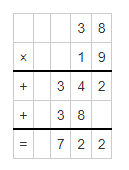Question 7.60 × 11 = 660.

Explanation:
Here, we have subtracted 1 from 11 and then multiplied 60 × 10 which is 600. Then added 60.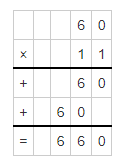Question 8.85 × 70 = 350.

Explanation:
Here, we have subtracted 5 from 85 and then multiplied 80 × 70 which is 5,600. Then subtracted 350.Question 9.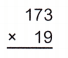173 × 19 = 3,287.

Explanation:
Here, we have added 1 to 19 and then multiplied 173 × 20 which is 3,460. Then subtracted 173.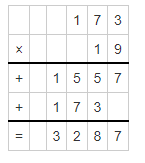Question 10.123 × 15 = 1,845.

Explanation:
Here, we have added 5 to 15 and then multiplied 123 × 20 which is 2,460. Then subtracted 615.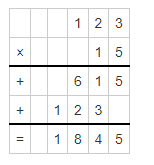Question 11.